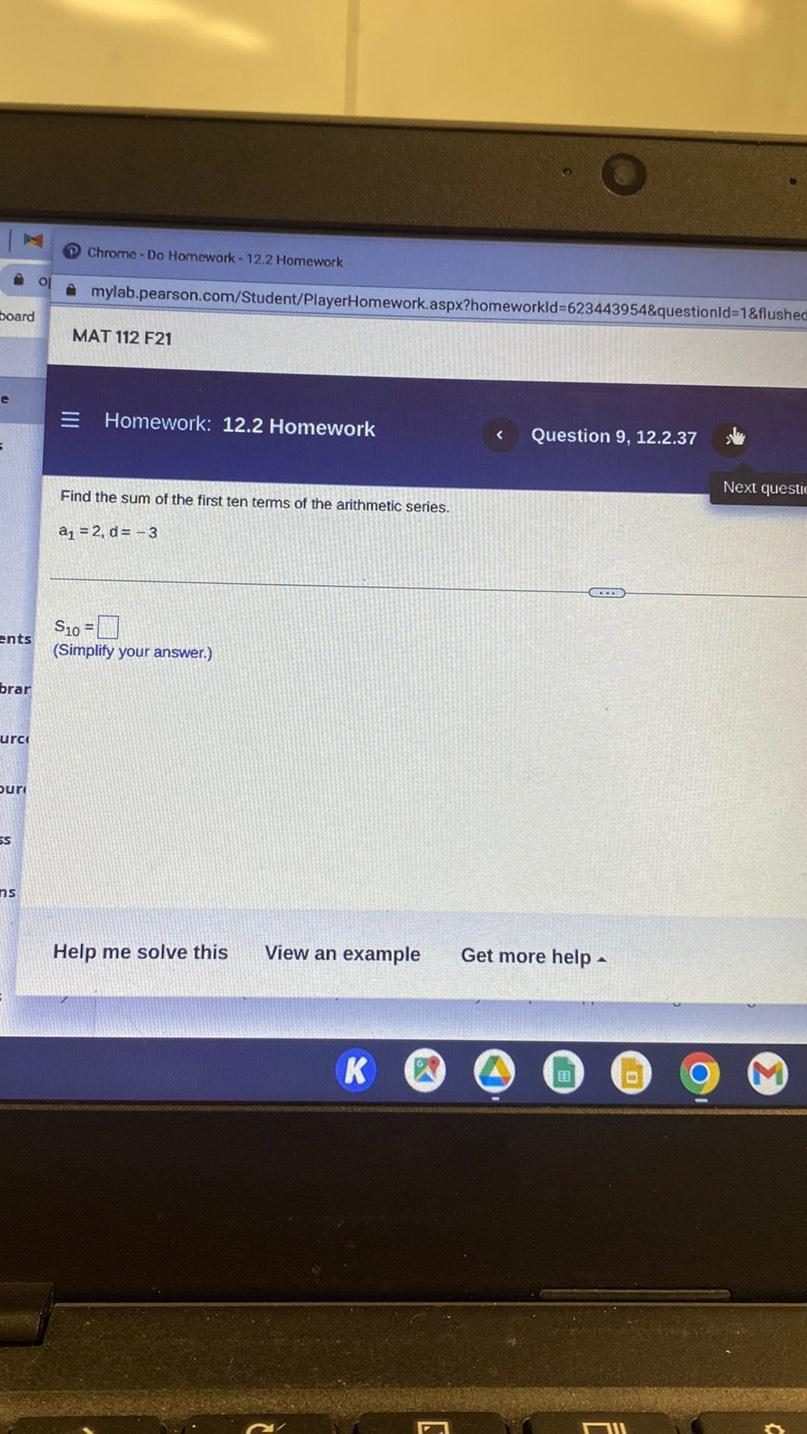Question:

# Find the sum of the first ten terms of the arithmetic series. a1 = 2, d = -3 S10 = ______.Find the sum of the first ten terms of the arithmetic series. a1 = 2, d = -3 S10 = ______.